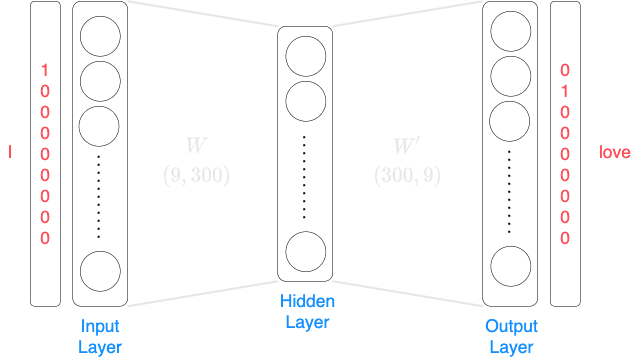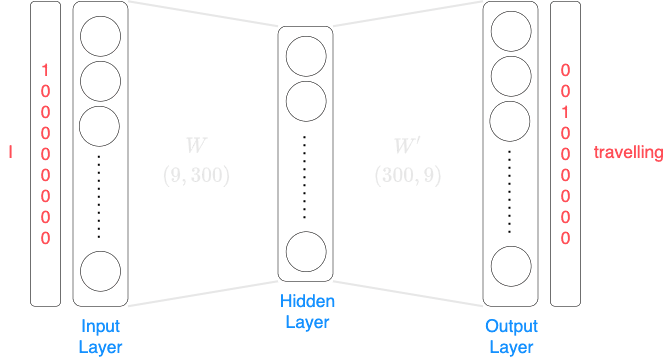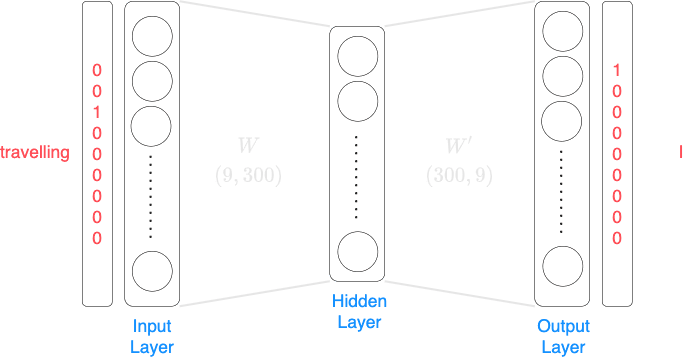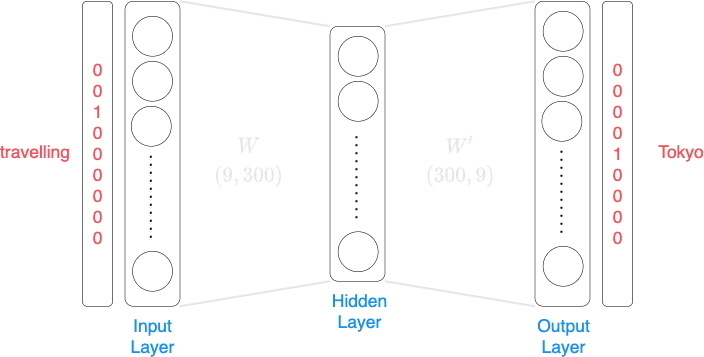search
Search
Guest 0reps
Thanks for the thanks!
close
Cancel
Post
account_circle
Profile
exit_to_app
Sign out
search
keyboard_voice
close
Searching Tips
Search for a recipe:
"Creating a table in MySQL"
Search for an API documentation: "@append"
Search for code: "!dataframe"
Apply a tag filter: "#python"
Useful Shortcuts
/ to open search panel
Esc to close search panel
to navigate between search results
d to clear all current filters
Enter to expand content previewDoc SearchCode Search BetaSORRY NOTHING FOUND!
mic
Start speaking...Voice search is only supported in Safari and Chrome.
Shrink
Navigate to
A
A
brightness_medium
share
arrow_backShareTwitterFacebook

# Comprehensive Guide on Word2Vec

Machine Learning
chevron_right
Neural Networks
schedule Jul 1, 2022
Last updated
local_offer Machine Learning
Tags
expand_more

Word2Vec obtains vector representations of words using either of the following two techniques:

• Continuous bag-of-words (CBOW)

• Skip-Gram

# Continuous bag-of-words (CBOW)

Consider the following text:

 I love travelling to Tokyo and eating Japanese food 

The objective of CBOW is to predict a word given its context, that is, its surrounding words. For instance, given the context ("love", "to"), we want to predict the word "travelling". The size of the context is known as the window size. If we take the window size to be 2, then the context of "travelling" becomes ("I", "love", "to", "Tokyo").

# Skip-Gram

The Skip-Gram approach is the inverse of CBOW, that is, the objective is to predict the context (surrounding words) given a word.

Once again, consider the following text:

 I love travelling to Tokyo and eating Japanese food 

Suppose the window size is 2, that is, we extract 2 words to the left and 2 words to the right of the input word.

Suppose the input word is the first word I. The corresponding targets would be as follows:

 lovetravelling 

Notice how there are no words to the left of the word I, and hence we only have 2 targets here. Here, we actually have two separate training data items to feed into the neural network:

• When the input is I, then the target label is love

• When the input is I, then the target label is travelling

For the first training data item in which the input is I and the target label is love, the neural network look the following:Note the following:

• the neural network is 2-layered, which means that there is only one hidden layer

• the number of neurons (or units) in the input layer corresponds to the size of the vocabulary, that is, the number of unique words in the corpus. In this case, the corpus is small and the vocabulary size is 9.

• the number of neurons in the output layer would also be the vocabulary size.

• the hidden layer consists of 300 neurons, that is, the weight matrix, $W$, from the input layer to the hidden layer would have 300 columns. After training, each row of the weight matrix $W$ corresponds to a vector representation of a word. This means that each word will be represented by a vector of size 300.

For the second training data item in which the input is I and the output is travelling, the neural network would look the following:Just as another example, suppose the input word is travelling. The corresponding targets would be as follows:

 IlovetoTokyo 

Here, we have a total of four separate training data items to feed into the neural network:

• When the input is travelling, then the target label is I

• When the input is travelling, then the target label is love

• When the input is travelling, then the target label is to

• When the input is travelling, then the target label is Tokyo

For the case when the input is travelling, and the target label is I, the neural network would be as follows:For the case when the input is travelling, and the target label is Tokyo, the neural network would be as follows:Can you see how just from a short sentence, we already end up with a large number of training data items. In a more practical scenario, the corpus that will be used to build the Word2Vec model is extremely large, and therefore the size of the training data set will be large as well.

# Word embedding

The matrix multiplication between the one-hot vector and the weight matrix results in simply knocking out one row of the weight matrix:

$$\begin{pmatrix} 0&1&0&0&0&0&0&0&0 \end{pmatrix}\cdot \begin{pmatrix} w_{1,1}&w_{1,2}&\cdots&w_{1,300}\\ \color{blue}w_{2,1}&\color{blue}w_{2,2}&\color{blue}\cdots&\color{blue}w_{2,300}\\ w_{3,1}&w_{3,2}&\cdots&w_{3,300}\\ w_{4,1}&w_{4,2}&\cdots&w_{4,300}\\ w_{5,1}&w_{5,2}&\cdots&w_{5,300}\\ w_{6,1}&w_{6,2}&\cdots&w_{6,300}\\ w_{7,1}&w_{7,2}&\cdots&w_{7,300}\\ w_{8,1}&w_{8,2}&\cdots&w_{8,300}\\ w_{9,1}&w_{9,2}&\cdots&w_{9,300}\\ \end{pmatrix} =\begin{pmatrix} \color{blue}w_{2,1}&\color{blue}w_{2,2}&\color{blue}\cdots&\color{blue}w_{2,300} \end{pmatrix}$$

The weight matrix $\mathbf{W}$ is known as distributed representation, or word embedding, of the words in the corpus. Interestingly, the “sense” of the words are captured, or encoded, by this distributed representation. For simplicity, suppose the window size is one. This means that we take one word to the left of the target, and one word to the right of the target. Each data item, then, would consist of two one-hot vectors. The big picture of our model is as follows:

mail